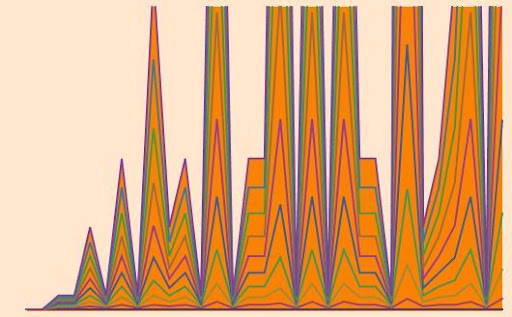## Monday, 21 December 2009

### SPLITTING FTA FUNCTIONS (II)

PILTZ DIVISOR FUNCTIONS (1)INTRO:

If we look for an example of "Functions that depend only on coefficients", our first idea should be the divisor function, $latex \tau_{2}(n)$ because it is multiplicative with:

$latex \tau_{2}(p^\alpha)=1+\alpha$

Here, they only appear the coefficients but not the primes.

With the help of recursive Dirichlet Convolution of the unit, $latex I_{0}(n)=1$, it is possible to construct a sequence of arithmetical functions only dependent on the coefficients of the prime factors of any number, known as Piltz Divisor Functions, $latex \tau_{k}(n)$, because they give the number of distinct solutions of the equation $latex x_{1}x_{2} \cdots x_{k}=n$, where $latex x_{1},x_{2},\cdots,x_{k}$ run indepently through the set of positive integers) or, if preferred, they give the number of ordered factorizations of $latex n$ as a product of $latex k$ terms. (References , and )

DEFINITION:

$latex \displaystyle \tau_{1}(n)=I_{0}(n)=1$

$latex \tau_{k}(n)=\sum_{d|n}^{}{\tau_{k-1}(d)\cdot I_{0}(n/d)}=\sum_{d|n}^{}{\tau_{k-1}(d)}$

This recursion can also be notated in terms of Dirichlet Product as:

$latex (f*g)(n)=\sum_{d|n}{f(d)\cdot g(n/d)}$

$latex \tau_{k}(n)=\tau_{k-1}*I_{0}(n)$

NOTES ON NOTATION:

The divisor function can be found on the literature as: $latex d(n)$, $latex \sigma_{0}(n)$, $latex \tau(n)$, and in this post as $latex \tau_{2}(n)$.

The "$latex \sigma$´s", and "$latex \tau$´s" are two different series of arithmetical functions that share one element in common: The divisor function. With the help of this two notations, it is possible to remark what kind of series we are working with.

On the other hand the "$latex d$", it is a simple notation that can be used for another purposes, were the belonging to this series of functions, does not matters.

Unfortunately, this happens not only with the divisor function, the mathematical notation on arithmetical functions related to Dirichlet convolution (or product) varies from one book to another, and not only distinct functions are named the same, but all cases of "non-biyectivity" between notations and functions can be found.

Hereinafter we are going to use:

$latex I_{k}(n)=n^{k}$ ( like in Reference  but with $latex I_{0}(n)=1$ and $latex I_{1}(n)=n$)

The identity element for Dirichlet´s product (or unit function), using Kronecker´s delta notation, is:

$latex \displaystyle \delta_{1n}= \bigg\lfloor \frac{1}{n} \bigg\rfloor$ (Reference )

$latex \omega(n)$ means the number of
distinct prime factors of $latex n$

PROPERTY:

$latex \tau_{k}(n)$ is multiplicative because $latex \tau_{k-1}(n)$, and $latex \tau_{1}(n)$ are multiplicative.

This property can also be derived from the behavior of the Dirichlet Product, but we must note that although $latex \tau_{1}(n)=I_{0}(n)=1$ is a completely multiplicative function, its convolution: $latex \tau_{2}(n)$ is multiplicative, but it is not completely multiplicative.

THEOREM-1:  and 

$latex \displaystyle \tau_{k}(n)=\prod_{i=1}^{\omega(n)}{\prod_{j=1}^{k-1}\frac{\alpha_{i}+j}{j}}=\prod_{i=1}^{\omega(n)}{\binom{\alpha_{i}+k-1}{k-1}}; \; (k \ge 1)$

Proof:

$latex \displaystyle \tau_{k}(p^\alpha)=\sum_{d|p^\alpha}^{}{\tau_{k-1}(d)}=\tau_{k-1}(1)+\tau_{k-1}(p)+\tau_{k-1}(p^2)+ \cdots +\tau_{k-1}(p^\alpha)$

$latex \displaystyle \tau_{k}(p^\alpha)= \sum_{i=0}^{\alpha}{\tau_{k-1}(p^i)} =\sum_{i=0}^{\alpha}{\binom{i+k-2}{k-2}=\binom{\alpha+k-1}{k-1}$

Lemma:

$latex \displaystyle \sum_{i=0}^{\alpha}{\binom{i+k-2}{k-2}}=\binom{\alpha+k-1}{k-1}$

Proof:

From Parallel Summation Identity (References  and ):

$latex \displaystyle \sum_{k=0}^{n}{\binom{k+r}{k}}=\binom{n+r+1}{n}$

Substituing: $latex \displaystyle n\rightarrow{\alpha}$ and $latex \displaystyle k\rightarrow{i}$

$latex \displaystyle \sum_{i=0}^{\alpha}{\binom{i+r}{i}}=\binom{\alpha+r+1}{\alpha}$

$latex \displaystyle r\rightarrow{k-2}$ and with Pascal´s Symmetry Rule :

$latex \displaystyle \sum_{i=0}^{\alpha}{\binom{i+k-2}{i}}= \sum_{i=0}^{\alpha}{\binom{i+k-2}{k-2}}= \binom{\alpha+k-1}{\alpha}=\binom{\alpha+k-1}{k-1}$

Corollary-1: Values of $latex \tau_{k}(s)$, being $latex s$ a squarefree number.

If $latex s$ is squarefree then all coefficients of its factorization are $latex \displaystyle \alpha_{i}(s)=1$, then:

$latex \displaystyle \tau_{k}(s)= \prod_{i=1}^{\omega(s)}{\binom{k}{k-1}=k^{\omega(s)}$

For a prime $latex p$, $latex \displaystyle \omega(p)=1$, and $latex \displaystyle \tau_{k}(p)=k$, and if $latex s=1$, then $latex \displaystyle \omega(1)=0$ and $latex \tau_{k}(1)=1$

Corollary-2:

$latex \displaystyle \tau_{k+1}(n^k)=\tau_{k}(n^k)\cdot \tau_2(n)$

Proof:

$latex \displaystyle \tau_{k+1}(n)=\tau_{k}(n)\cdot \prod_{i=1}^{\omega(n)}{\frac{\alpha_{i}+k}{k}}$

$latex \displaystyle \tau_{k+1}(n^k)=\tau_{k}(n^k)\cdot \prod_{i=1}^{\omega(n^k)}{\frac{\alpha_{i}+k}{k}}$

Like $latex \displaystyle \omega(n^k)=\omega(n)$, and $latex \displaystyle \alpha_{i}(n^k)=k\cdot\alpha_{i}(n)$:

$latex \displaystyle \tau_{k+1}(n^k)=\tau_{k}(n^k)\cdot \prod_{i=1}^{\omega(n)}{\frac{k \cdot \alpha_i+k}{k}}=\tau_{k}(n^k)\cdot \prod_{i=1}^{\omega(n)}{(\alpha_i+1)}=\tau_{k}(n^k)\cdot \tau_2(n)$

References:

-p.167-Exercise 5.b - Leveque, William J. (1996) . Fundamentals of Number Theory. New York: Dover Publications. ISBN 9780486689067
-T. M. Apostol, Introduction to Analytic Number Theory, Springer-Verlag, 1976, pages 29 and 38
-J. Sándor: On the Arithmetical Functions $latex d_{k}(n)$ and $latex d_{k}^{*}(n)$, Portugaliæ Mathematica 53, No. 1 (1996)
-I.Vinogradov, Fundamentos de la Teoría de los Números, Editorial RUBIÑOS, ISBN 84-2222-210-8, Segunda Edición,chapter II, Exercise 11, page 44
-J. Sándor, B. Crstici, Handbook of Number Theory (Vol II), Kluwer Academic Publishers, Springer, 2004 ISBN 1402025467, 9781402025464
-Ken.J.Ward, Ken Ward's Mathematics Pages, Parellel Summation-Formula 2.2.2
-Matthew Hubbard and Tom Roby - Pascal's Triangle From Top To Bottom -Catalog #: 1000001
-Matthew Hubbard and Tom Roby - Pascal's Triangle From Top To Bottom - Catalog #: 1100002
-A000012-The simplest sequence of positive numbers: the all 1's sequence. The On-Line Encyclopedia of Integer Sequences!
-A000005-d(n) (also called tau(n) or sigma_0(n)), the number of divisors of n. The On-Line Encyclopedia of Integer Sequences!
-A007425-d_3(n), or tau_3(n), the number of ordered factorizations of n as n = rst. The On-Line Encyclopedia of Integer Sequences!